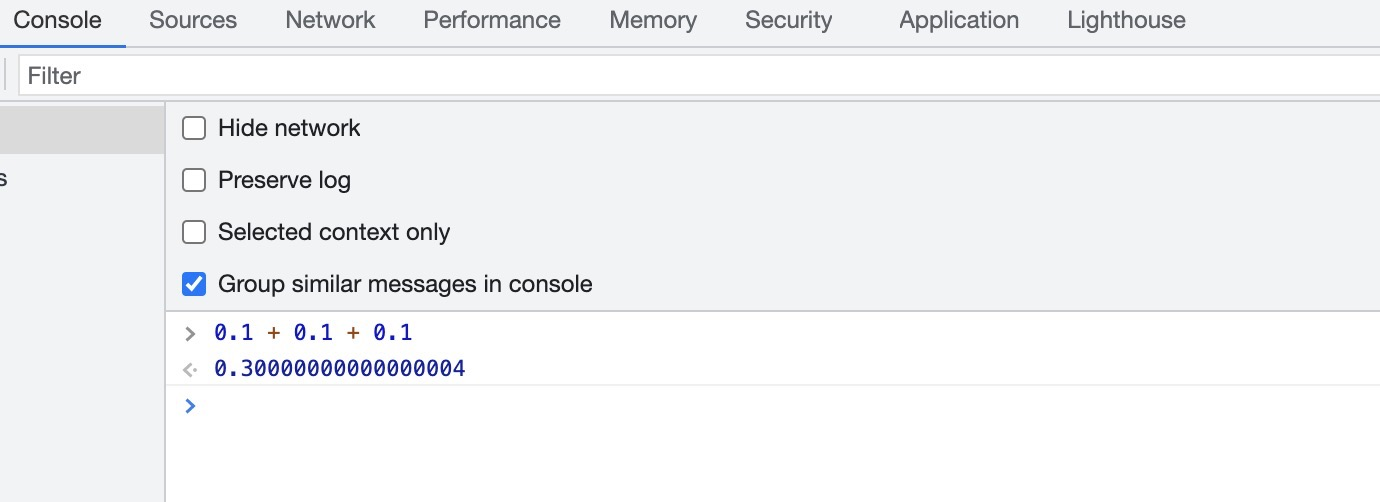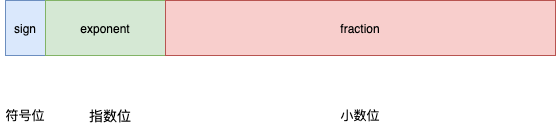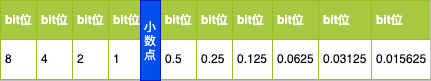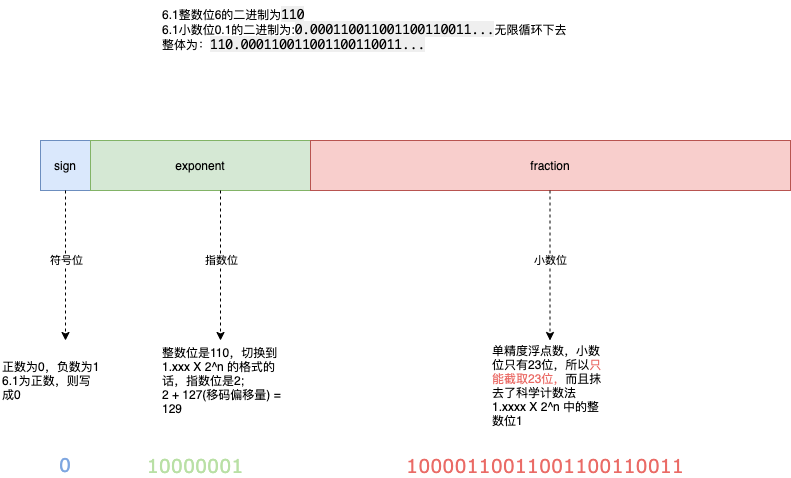## golang浮点数精度丢失问题详解

,
0 评论0 浏览

``````var num float32
for i := 0; i < 7; i++{
num = num + 0.1
}

fmt.Println(num)
``````

OK，我们换JS试试。`把一个数表示成a与10的n次幂相乘的形式（1≤|a|<10，a不为分数形式，n为整数），这种记数法叫做科学记数法。```````0.1 X 2 = 0.2  0
0.2 X 2 = 0.4  0
0.4 X 2 = 0.8  0
0.8 X 2 = 1.6  1
(1.6 - 1 = 0.6)
0.6 X 2 = 1.2  1
(1.2 - 1 = 0.2)
0.2 X 2 = 0.4  0
0.4 X 2 = 0.8  0
0.8 X 2 = 1.6  1
(1.6 - 1 = 0.6)
0.6 X 2 = 1.2  1
(1.2 - 1 = 0.2)
0.2 X 2 = 0.4  0
0.4 X 2 = 0.8  0
0.8 X 2 = 1.6  1

...
// 无限循环下去
``````

OK，看来小数位的数可以确定了是`10000110011001100110011`，即去掉整数位1后，向后截取的23位数(浮点数不精确的本质原因)。

``````例如：1.01 X 2^-1 和 1.11 X 2^3比较大小？

首先对比指数位， -1 和 3，分别转化为二进制数 ``111``和``011``；

如果没有其他逻辑处理，``111``是"7"，``011``是"3"， 7会小于3吗？
``````

``````例如：1.01 X 2^-1 和 1.11 X 2^3比较大小？

指数为-1的则表示为 -1 + 4 = 3，二进制表示为:011

指数为3的则表示为 3 + 4 = 7 二进制表示为：111

7 > 3，即 111 > 011 比较完毕
``````

``````假设偏移量是：4

``````

`IEEE 754`中，指数位移码的偏移量为指数位数的 `2^n-1 - 1`，为127。